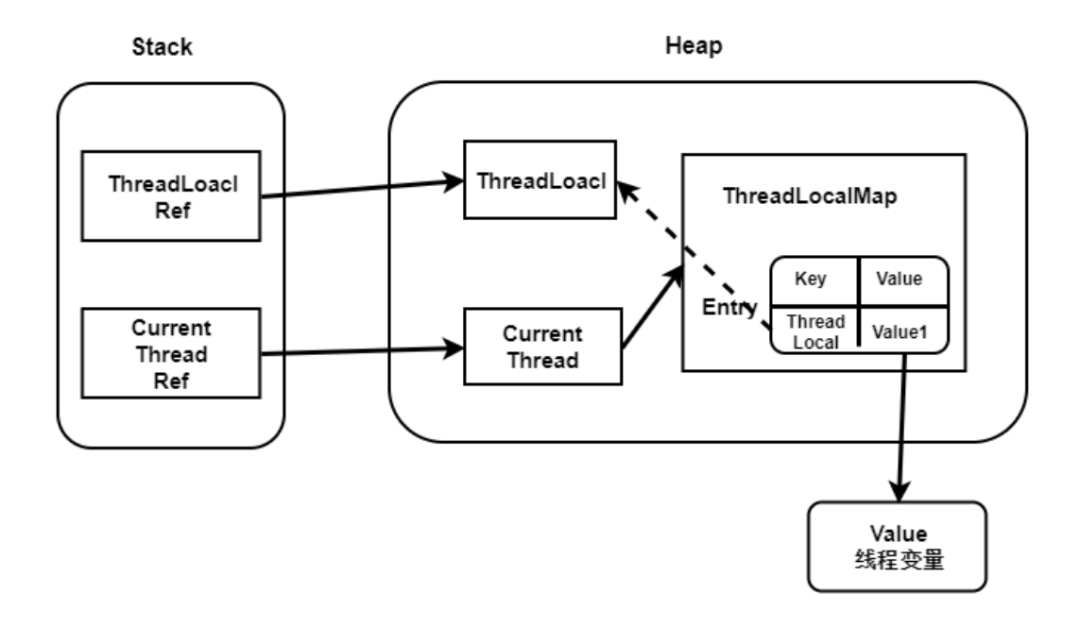maxdl

0收藏

### 什么是内存泄露``````private ThreadLocalMap(ThreadLocalMap parentMap) {
Entry[] parentTable = parentMap.table;
int len = parentTable.length;
setThreshold(len);
table = new Entry[len];

for (int j = 0; j < len; j++) {
Entry e = parentTable[j];
if (e != null) {
@SuppressWarnings("unchecked")
if (key != null) {
Object value = key.childValue(e.value);
Entry c = new Entry(key, value);
int h = key.threadLocalHashCode & (len - 1);
while (table[h] != null)
h = nextIndex(h, len);
table[h] = c;
size++;
}
}
}
}``````

### 弱引用

JVM进行垃圾回收时，无论内存是否充足，都会回收被弱引用关联的对象。在 java 中，用 java.lang.ref.WeakReference 类来表示。可以在缓存中使用弱引用。``````static class ThreadLocalMap {
static class Entry extends WeakReference<ThreadLocal<?>> {
Object value;
super(k);
value = v;
}
}

**成员变量**
//初始容量
private static final int INITIAL_CAPACITY = 16;

private Entry[] table;

private int size = 0;

//达到这个大小，则扩容
private int threshold; ``````

### 构造函数

``````ThreadLocalMap(ThreadLocal<?> firstKey, Object firstValue) {
//初始化table数组，INITIAL_CAPACITY默认值为16
table = new Entry[INITIAL_CAPACITY];
//key和16取得哈希值
int i = firstKey.threadLocalHashCode & (INITIAL_CAPACITY - 1);
//创建节点，设置key-value
table[i] = new Entry(firstKey, firstValue);
size = 1;
//设置扩容阈值
setThreshold(INITIAL_CAPACITY);
}``````

``````private void remove(ThreadLocal<?> key) {
Entry[] tab = table;
int len = tab.length;
int i = key.threadLocalHashCode & (len-1);
for (Entry e = tab[i];
e != null;
e = tab[i = nextIndex(i, len)]) {
//如果key相同
if (e.get() == key) {
//删除当前Entry
e.clear();
//清理
expungeStaleEntry(i);
return;
}
}
}``````